# Dueling Dilettantes

[This is post #4 in a series on the Thue-Morse sequence. See posts #1, #2, and #3.]

What? You don’t agree that the Thue-Morse sequence is awesome? OK, there’s only one way to settle this dispute: I challenge you to a duel. This unfortunately might take a while, because we both have terrible aim.

Let’s say we both have the same probability $$p=.2$$ of hitting our target on any given shot, and the first to hit is the winner. I’ll even be gracious and let you go first, and I’ll shoot second. In the first two shots of the game, the probability that you win (i.e., hit your first shot) is $$p=.2$$, and the probability that I win (i.e., you miss and then I hit) is $$(1-p)\cdot p = .16$$. (In the remaining probability $$1-.2-.16=.64$$, the duel continues.) This means that you are more likely to win during the first two shots than I am, so it should only be fair that I take the third shot!

How do the probabilities look in the first three shots? Your chances of winning are still $$p=.2$$, while mine are $$(1-p)\cdot p + (1-p)^2\cdot p = .288$$ because I might win on the second or third shot. Now it seems that I have the advantage, so you should take the 4th shot in the interest of fairness. Let’s continue like this, choosing to give the 5th shot to the theoretical underdog from the first 4 rounds, the 6th shot to the underdog in the first 5 rounds, and so on.

You can (and should!) check that the shot order will be Y, M, M, Y, M, Y, Y, M, M, Y,  M, Y, Y, M, M, Y, ..., where Y=You and M=Me. Does that sequence look familiar? The first 10 digits look suspiciously like the start of the Thue-Morse sequence, but I don’t recognize the rest of it. Did we make a computation error? Hmm…

The problem, as it turns out, is that our aim is too good! What happens if we make ourselves worse by, say, standing farther apart? Let’s say we now have a $$p=.1$$ chance of hitting our mark on each shot. What is the “fair” shot order this time? Our duel will now follow the sequence Y, M, M, Y, M, Y, Y, M, M, Y, Y, M, Y, M, M, Y, M, Y, Y, M,  M, Y, Y, ... which matches the Thue-Morse sequence in the first 20 digits. Similarly, setting $$p=.05$$ gives 48 correct Thue-Morse digits, defining $$p=.01$$ gives 192 correct Thue-Morse digits, and decreasing to $$p=.001$$ gives 1536 matching digits! It can in fact be proved that decreasing $$p$$ toward 0 (i.e., making our aim worse) gives more and more Thue-Morse digits, with the full Thue-Morse sequence appearing in the limit.

See? Even when arguing about whether the Thue-Morse sequence is cool, we find evidence of its greatness! I hereby declare myself the winner of this duel.

This concludes our series on the Thue-Morse sequence. Tune in next week for, well, something else!

### Notes

1. Joshua Cooper and Aaron Dutle. Greedy Galois Games. ArXiv, 2011. []

# Chess, Bored

[This is post #3 in a series on the Thue-Morse sequence, which was introduced two weeks ago and discussed further last week.]

While the Thue-Morse sequence appears in many branches of Mathematics, this sequence has also captured a place in the history of chess.

With only the basic rules of chess (specifically, how pieces move and capture and how a game may end by checkmate or stalemate), there is no guarantee that a game of chess will ever finish. For example, two (very bored!) players could theoretically move their knights back and forth ad infinitum without ever engaging each other or capturing a piece (but why would they want to?). A more realistic example is drawn below. In this game, black will soon checkmate white if given the freedom to move, and white has no way to win. However, white can avoid losing by keeping black in an unending runaround with repeated checks, as illustrated. In this case (a phenomenon known as perpetual check), forcing an infinite game is more advantageous for white than allowing an otherwise inevitable loss.

Because of this possibility of never finishing, a search began for new chess rules to guarantee that every game of chess eventually ends. One suggested rule was:

A chess game ends with a draw if a sequence of moves—with all pieces in exactly the same positions—is played three times successively.

The hope was that any sufficiently long chess game would eventually repeat itself enough to break this rule. However, as Mathematician and Chess Grandmaster Max Euwe showed in 1929, this hope is not correct. Indeed, there exist infinite chess games that never repeat the same block of moves three times in a row!

How did he prove this? With the Thue-Morse Sequence, of course! He proved the very unintuitive fact that the Thue-Morse sequence has no chunk repeated three times consecutively. Such a thrice-repeated sequence is called a cube, so this property can be restated by saying that the Thue-Morse sequence is cube-free. For example, you will never find the sequences 1 1 1 or 011 011 011 in the Thue-Morse sequence. (Challenge: show that these two sequences do not appear. Harder challenge: show that the Thue-Morse sequence is cube free!) So, if the “very bored” knight-flippers described above decide to move their right knights or left knights according to the 1s and 0s of the Thue-Morse sequence, the resulting game will have no tripled move sequences.

Luckily, the above rule is not part of the current official chess rule set. There are now two rules on the books that can be used to eventually end any chess game. (In fact, either one would suffice. [Prove this!]) A draw may be declared in either of these situations:

• The exact same board position appears three times ever during a game. (Every detail must be the same, such as whose turn it is, which kings have the ability to castle, etc.)
• No pawn is moved and no piece is captured in 50 consecutive turns. (A turn consists of one move by each player.)

However, there’s (always) a catch. These two rules only take effect if a player notices and decides to declare a draw. So even with all modern rules in effect, the bored knight-flippers are still allowed to play their infinite game of chess—cube-free or not.

### Notes

1. A great survey called The ubiquitous Prouhet-Thue-Morse sequence by Allouche and Shallit documents many sightings of this sequence throughout Mathematics, including Combinatorics, Differential Geometry, Number Theory, Analysis, and as discussed here, Combinatorial Game Theory. []
2. Manfred Börgens, Max Euwe’s sequence []
3. in his paper titled Mengentheoretische Betrachtungen über das Schachspiel []

# Thue-Morse-igami

Here’s a simple origami experiment to try. Start with a long strip of paper with different colors on the two sides—e.g., Orange (O) on one side, Indigo (I) on the other. Now fold the strip in quarters to form a flat “S-shape” as illustrated here (creases are drawn slightly rounded for ease of viewing):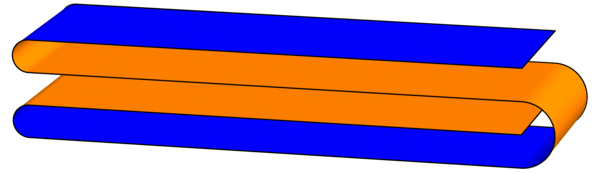As you look through the four layers from top to bottom, write down the colors you encounter, in order: the first layer shows Indigo (I), the second layer has Orange (O), and so on. The full order is IOOI, which looks suspiciously like the start of the Thue-Morse sequence. (This sequence was introduced last week.)

To continue the experiment, treat this figure as a single strip and fold the same flat S-shape again. There will be 16 layers down the middle: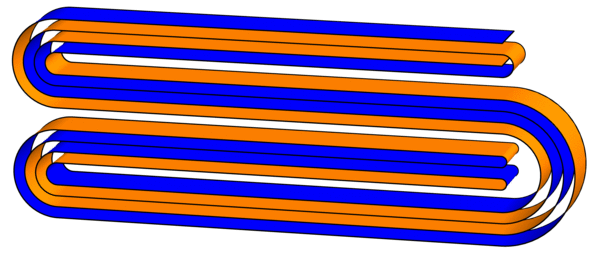Sure enough, the order of colors encountered is IOOI OIIO OIIO IOOI, which is the first 16 digits of the Thue-Morse sequence. This pattern will continue forever (or at least until the paper gets too thick to fold): every time you fold another S-shape, you quadruple the number of digits of the Thue-Morse sequence (can you see why?). Try to fold a third iteration! It looks like this (click for larger image):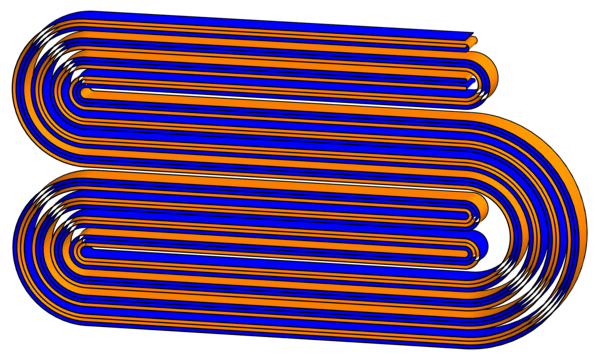But wait, there’s more! Now that we have these creased strips, what happens when we unfold them? Specifically, follow the existing creases and lay out the strips so that each crease makes a 90-degree angle. What shapes do we get? Surprisingly, they exactly fill triangular grids of squares:Can you figure out why this pattern continues?

# Thue-Morse Navigating Turtles

Here’s a simple rule to generate a pretty sequence of 1s and 0s: Start with the list 1 (just one digit) and repeatedly form a new list by flipping all the bits (1s become 0s and vice versa) and attaching this to the end: $$1 \to 10 \to 1001 \to 10010110 \to 1001011001101001 \to \cdots.$$ For example, to get from 1001 to the next sequence, we swap 1s and 0s to form 0110, and then we join these together to make 10010110. By continuing this process we can make our list longer and longer, and the limiting sequence $$1001011001101001011010011001011001101\cdots$$ is called the Thue-Morse Sequence.

What did I mean by calling this sequence “pretty”? Well, what does this sequence “look like”? One way to visualize it is with a turtle program. Imagine a turtle (let’s call him Leo) walking along the plane, and treat the digits of the Thue-Morse sequence as a list of instructions for the turtle as follows: When Leo sees the digit 1, he steps forward one foot; a 0 tells Leo to turn left by 60 degrees. What does Leo’s path look like? Here are the first 32 instructions he follows: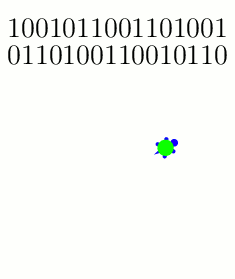The turtle follows these instructions on the first 32 entries of the Thue-Morse sequence: {1: Step; 0: Turn 60}.

And here are snapshots of his path as he gets farther into the Thue-Morse sequence: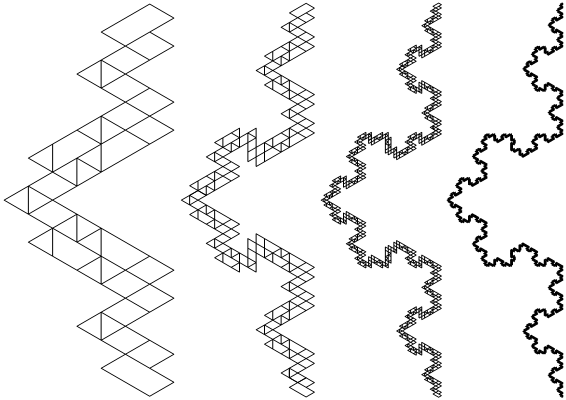Snapshots of the turtle's trajectories after 2^8, 2^10, 2^12, and 2^14 instructions, respectively.

Why did we pick these instructions? What if Leo turns 120 degrees instead of 60 degrees when he sees a 0?The turtle follows the Thue-Morse sequence with a slightly different set of instructions: {1: Step; 0: Turn 120}. Shown here after 2^6, 2^8, 2^10, and 2^12 instructions, respectively.

If you zoom out and/or squint a little, these paths are roughly following a famous fractal called the Koch curve. And indeed, the more steps you draw, the closer the resemblance will become (as analyzed in these two papers: [MH2005] and [KZH2008]). But why stop here? What happens if we change the turtle’s instructions even more? I played around with some new rule sets, and these too show how the Koch curve seems innately “programmed” into the structure of the Thue-Morse sequence:Three more pretty examples of the Thue-Morse sequence's "turtle power." In all three cases, the trajectories converge to the Koch curve. Left: {1: (Turn 180, Step); 0: (Turn 60, Step)}, after 2^8 instructions. Middle: {1: (Turn 180, Step); 0: (Turn 120, Step)}, after 2^10 instructions. Right: {1: Turn 180; 0: (Turn 15, Step)}, after 2^10 instructions.

Here’s a final challenge: if the Koch curve and the Thue-Morse sequence are so intimately related, shouldn’t we be able to find some turtle program instructions that trace the Koch curve without “squinting”? Specifically, the Koch curve is usually constructed as the limit of the following sequence of paths, where each iteration is made by stitching together four copies of the previous curve:The Koch curve is constructed as the limit of these paths, where each curve is formed from four (shrunken) copies of the previous curve.

Is there a set of turtle instructions whose path traces exactly these curves? In fact, there is! These instructions work: {1: (Step, Turn 60); 0: Turn 180}.

# Astounding “Natural” Identities

The modest sequence $$1,2,3,4,\ldots$$ can do some rather awe-inspiring things, when properly arranged. Here’s a short list of some of its many impressive feats.

There are numerous expressions for $$\pi$$ relying on the progression of integers, including the Wallis formula: $$\frac{\pi}{2} = \frac{2}{1} \cdot \frac{2}{3} \cdot \frac{4}{3} \cdot \frac{4}{5} \cdot \frac{6}{5} \cdot \frac{6}{7} \cdots$$ (which can be derived from Complex Analysis using an infinite product representation for the sine function) and an elegant alternating sum: $$\frac{\pi-3}{4} = \frac{1}{2\cdot 3\cdot 4}-\frac{1}{4\cdot 5\cdot 6}+\frac{1}{6\cdot 7\cdot 8}-\cdots$$ (try to prove this!). Euler’s number $$e$$ has similarly surprising formulas, such as $$\frac{1}{e-2} = 1+\frac{1}{2+\frac{2}{3+\frac{3}{4+\frac{4}{5+\cdots}}}}$$ (listed at MathWorld) and $$\frac{e}{e-1} = 1+\frac{1+\frac{1+\frac{1+\cdots}{2+\cdots}}{2+\frac{2+\cdots}{3+\cdots}}}{2+\frac{2+\frac{2+\cdots}{3+\cdots}}{3+\frac{3+\cdots}{4+\cdots}}}$$ (which is problem 1745 in Mathematics Magazine, posed by Gerald A. Edgar).

The list doesn’t stop here! The nested square-root identity $$3 = \sqrt{1+2\sqrt{1+3\sqrt{1+4\sqrt{1+\cdots}}}}$$ is attributed to Ramanujan (on this Wikipedia page). As another curiosity, the sequence $$\frac{1}{2},\qquad \frac{1}{2} \Big/ \frac{3}{4},\qquad \frac{\frac{1}{2} \big/ \frac{3}{4}}{\frac{5}{6} \big/ \frac{7}{8}},\qquad \frac{\frac{1}{2} \big/ \frac{3}{4}}{\frac{5}{6} \big/ \frac{7}{8}} \bigg/ \frac{\frac{9}{10} \big/ \frac{11}{12}}{\frac{13}{14} \big/ \frac{15}{16}},\qquad\ldots$$ (which relates to the Thue-Morse sequence) can be shown to converge to $$\sqrt{2}/2$$.

There are many pretty/unexpected/crazy formulas obtainable from the natural numbers $$1,2,3,4,\ldots$$ that could not fit in this post. What are some of your favorites?